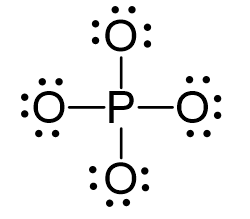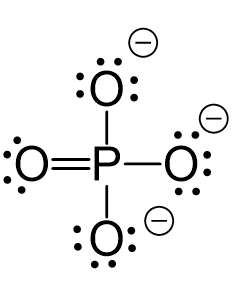## Examples

Phosphorous is the central and there are 5 + 4×6 + 3 = 32 electrons. Give each oxygen three lone pairs:All the electrons are used and the only thing to fix is the ionic charge. As drawn, it would be a -4, therefore, we give one lone pair from an oxygen to make a double bond with the P which can exceed the octet:The central atom has four atoms and no lone pairs, therefore, both geometries are tetrahedral.Steric number 4 corresponds to sp3-hybridization where the idealized bond angles are 109.5o.